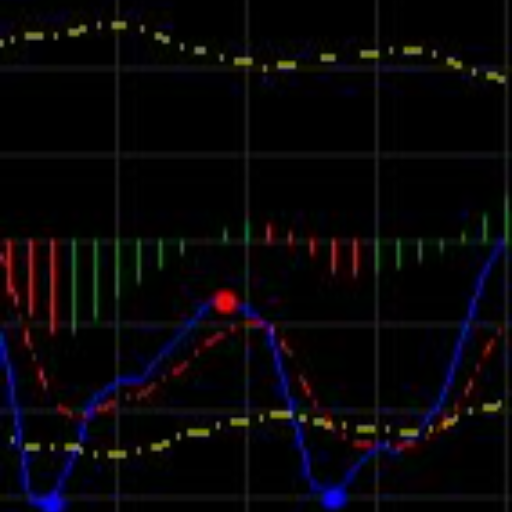Category Trend  at 08/11/2023

# Rolling VWAP

##### Description

Similar with VWAP, but instead of reset at an anchor point, the calculation is kept rolling, in a similar way that moving average is calculated.

I found it is somewhat interesting, it looks similar to Bollinger Band, but there is differences. The main line is the VWAP instead of ma. So I put it in Trend, although you can spot Volarity.

You can spot some squeeze period before a boost. Please share your usage!

Note that there is a difference in the way I calculated std versus the Bollinger Band:

• Bollinger Band: the deviation of each xi = (xi - mean at latest) . They assumes a straight line from the latest mean.
• This approach: the deviation of each xi = (xi - mean i).

This result in a smoother in the band.

Parameters:

• Period: the number of bars used for calculation.
• StdFactor: factor used for calculating the bands.

PS: For enquiries please drop me an email. (I answered a comment on this platform and got blocked by accident, may be by their auto bot).

The source code is almost fully published.

``````
using System;
using System.Collections.Generic;
using System.Linq;
using System.Text;
using cAlgo.API;
using cAlgo.API.Collections;
using cAlgo.API.Indicators;
using cAlgo.API.Internals;

namespace cAlgo
{
[Indicator(IsOverlay = true, AutoRescale = true, AccessRights = AccessRights.None)]
public class LT_Ind_RollingVWAP : Indicator
{
[Parameter(DefaultValue = 20)]
public int Period { get; set; }

[Parameter(DefaultValue = 3.0)]
public double StdFactor { get; set; }

[Output("UpBand", LineColor = "Red", LineStyle = LineStyle.Dots)]
public IndicatorDataSeries UpBand { get; set; }
[Output("Vwap", LineColor = "Orange")]
public IndicatorDataSeries Vwap { get; set; }
[Output("DnBand", LineColor = "Blue", LineStyle = LineStyle.Dots)]
public IndicatorDataSeries DnBand { get; set; }

IndicatorDataSeries _sqrDiff;
protected override void Initialize()
{
_sqrDiff = CreateDataSeries();
}

public override void Calculate(int index)
{
// The vwap at index
var list = Bars.Rev(index).Take(Period).ToList();
if (list.Count <= 0 || list.Last().TickVolume == 0) return;
var vwap = list.Sum(x => x.TickVolume * (x.High + x.Low + x.Close) / 3) / list.Sum(x => x.TickVolume);
Vwap[index] = vwap;

// Square diff at index (Use close instead of hlc3 for more variation)
_sqrDiff[index] = (Bars.ClosePrices[index] - vwap).MathPow(2);

// Std at index
var sqdList = _sqrDiff.Rev(index).Take(Period).ToList();
var std = sqdList.Sum().MathSqrt() / sqdList.Count;
UpBand[index] = vwap + StdFactor * std;
DnBand[index] = vwap - StdFactor * std;
}

}

}
```
```##### dhnhuy

Joined 03.04.2023

• Type: Free
• Language: C#